In: Physics

# Salaries for teachers in a particular elementary school district are normally distributed with a mean of...

Salaries for teachers in a particular elementary school district are normally distributed with a mean of $46,000 and a standard deviation of$4,900. We randomly survey ten teachers from that district. (Round your answers to the nearest dollar.)

(a) Find the 90th percentile for an individual teacher's salary.

(b) Find the 90th percentile for the average teacher's salary.

## Solutions

##### Expert Solution

let,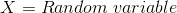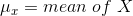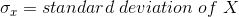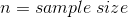if a random sample of size n is drawn from the population, then as n increases, the random variable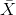which consists of sample means, tends to be normally distributed and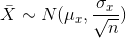According to central limit theorem for sample means or averages, when larger and larger samples are drawn from the population and their means are calculated, it is found that the sample means form their own normal distribution and the normal distribution has mean same as the original distribution and the variance equal to original variance divided by n.

(note: variance is the square of standard deviation)

The random variablehas different values of z scores associated with it, which is given as follows: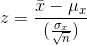where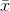is the value ofin one sample

Note that,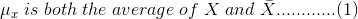whereas,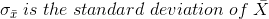and is given by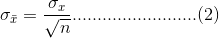a) For an individual teacher's salary, the sample size is 1

The mean and standard deviation for the distribution are: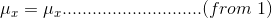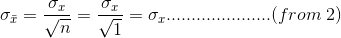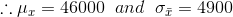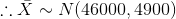Now for calculating the 90th percentile, we use NORM.INV function of Excel which has format NORM.INV(probability,mean,standard deviation)

Here, we have to calculate the 90th percentile for an individual teacher's salary, which indicates the salary below which 90% of the individual teachers fall.

Thus we have to find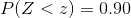we make the use of excel for finding value of Z

thus using NORM.INV(probability,mean,standard deviation) = NORM.INV(0.90,46000,4900), we get the required value of salary as 52280 i.e $52,280 Thus the 90th percentile for individual teacher's salary is$ 52,280

b) For the average teacher's salary, the sample size is 10

Thus the mean and standard deviation for the distribution are: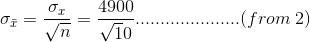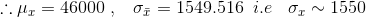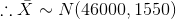Now using NORM.INV function of Excel to calculate 90th percentile as in part a),

NORM.INV(probability,mean,standard deviation) = NORM.INV(0.90,46000,1550) we get required value of salary as 47986 i.e $47,986 Thus the 90th percentile for the average teacher's salary is$ 47,986.

## Related Solutions

##### Salaries for teachers in a particular elementary school district are normally distributed with a mean of...
Salaries for teachers in a particular elementary school district are normally distributed with a mean of $44,000 and a standard deviation of$6,500. We randomly survey ten teachers from that district. 1.Find the probability that the teachers earn a total of over $400,000 2.If we surveyed 70 teachers instead of ten, graphically, how would that change the distribution in part d? 3.If each of the 70 teachers received a$3,000 raise, graphically, how would that change the distribution in part...
##### Salaries for teachers in a particular elementary school district are normally distributed with a mean of...
Salaries for teachers in a particular elementary school district are normally distributed with a mean of $44,000 and a standard deviation of$6,500. We randomly survey ten teachers from that district. Find the 85th percentile for the sum of the sampled teacher's salaries to 2 decimal places.
##### A researcher claims that the mean of the salaries of elementary school teachers is greater than...
A researcher claims that the mean of the salaries of elementary school teachers is greater than the mean of the salaries of secondary school teachers in a large school district. The mean of the salaries of a random sample of 26 elementary school teachers is $48,250 and the sample standard deviation is$3900. The mean of the salaries of 24 randomly selected secondary school teachers is $45,630 with a sample standard deviation of$5530. At ? = 0.05, can it...
##### A study was conducted to estimate the difference in the mean salaries of elementary school teachers...
A study was conducted to estimate the difference in the mean salaries of elementary school teachers from two neighboring states. A sample of 10 teachers from the Indiana had a mean salary of $28,900 with a standard deviation of$2300. A sample of 14 teachers from Michigan had a mean salary of $30,300 with a standard deviation of$2100. Determine a 95% confidence interval for the difference between the mean salary in Indiana and Michigan.(Assume population variances are different.) *Include...
##### A researcher claims that the variation in the salaries of elementary school teachers is greater than...
A researcher claims that the variation in the salaries of elementary school teachers is greater than the variation in the salaries of secondary school teachers. A random sample of the salaries of 30 elementary school teachers has a variance of $8,208, and a random sample of the salaries of 30 secondary school teachers has a variance of$3,817. At α = 0.05, can the researcher conclude that the variation in the elementary school teachers’ salaries is greater than the variation...